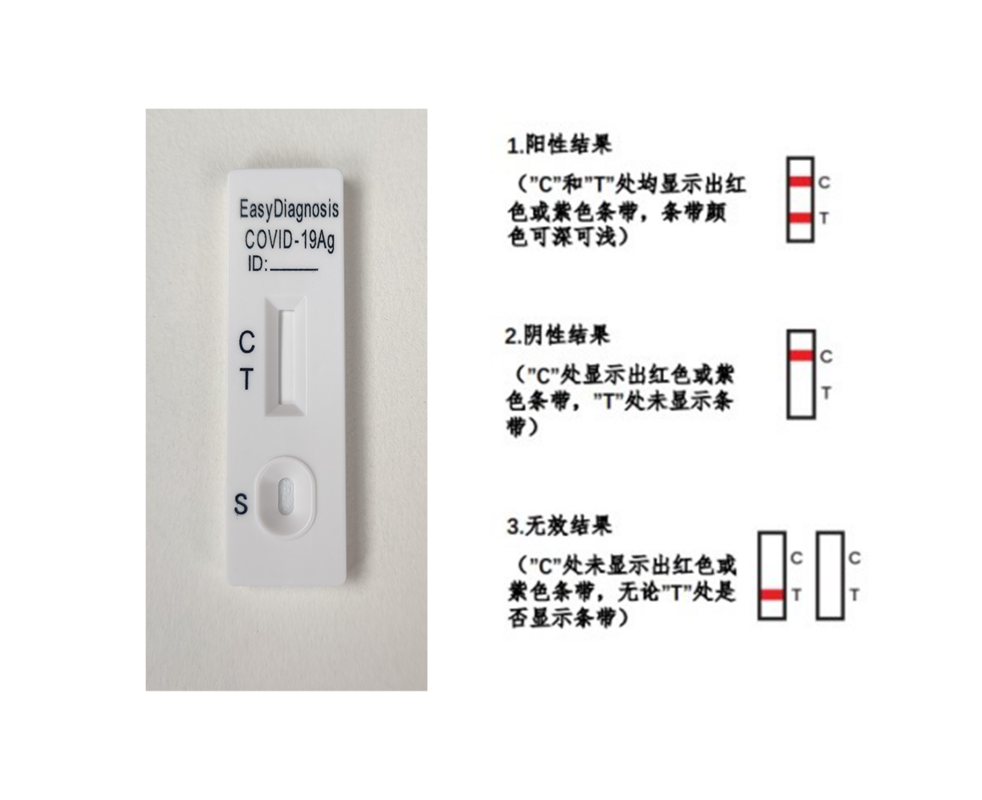TOP龙虎榜

TOP魅力榜

TOP火花榜

# 抗原检测出阳性？莫慌！37 人参与了问题讨论p(~B|A)=p(A|~B)p(~B)/p(A)=(1-p(~A|~B))(1-p(B))/(p(A|B)p(B) + (1-p(~A|~B))(1-p(B))) = 0.010.97 / (0.950.03 + 0.01*0.97) = 0.2539

• 1
• 0
• 评论(1)
• 举报